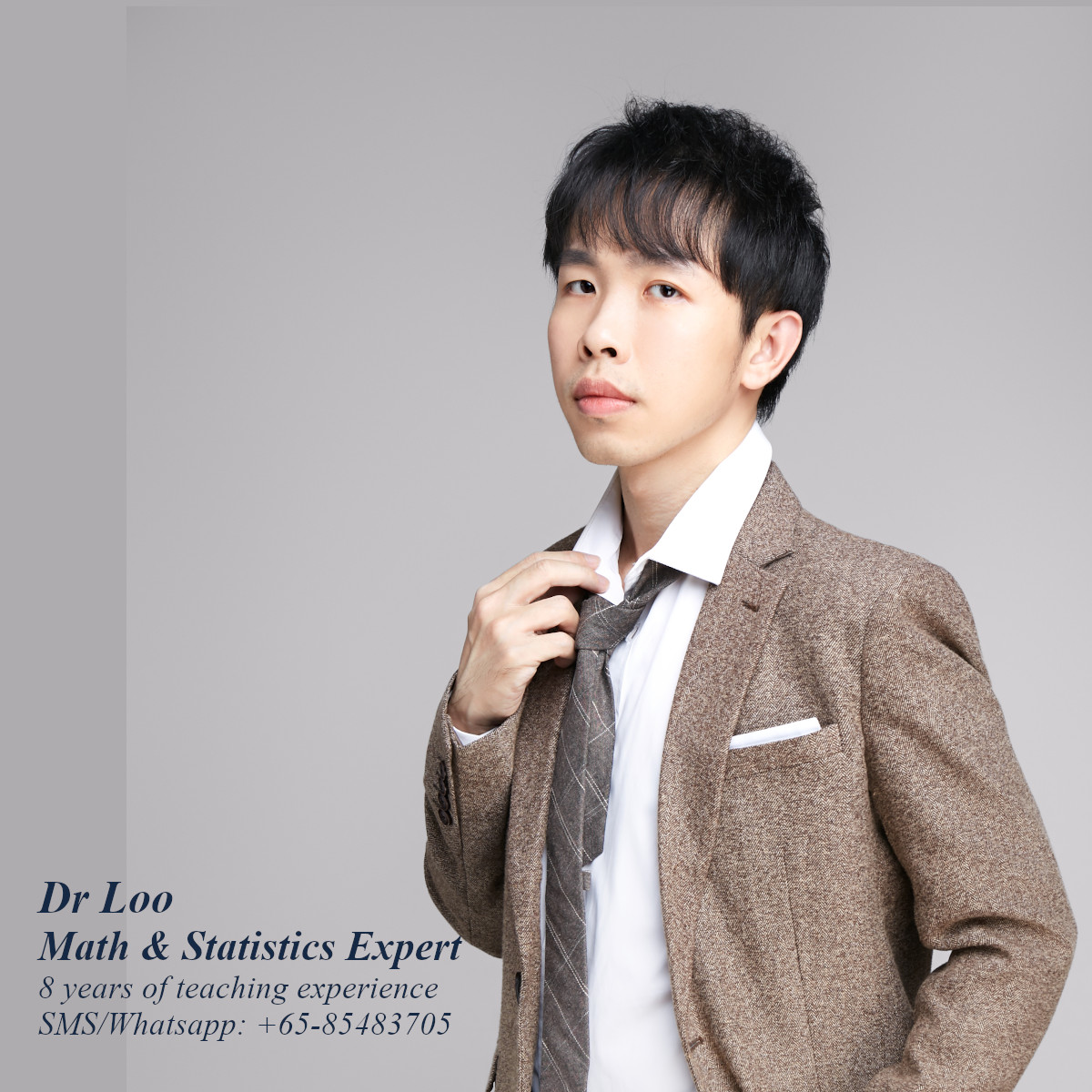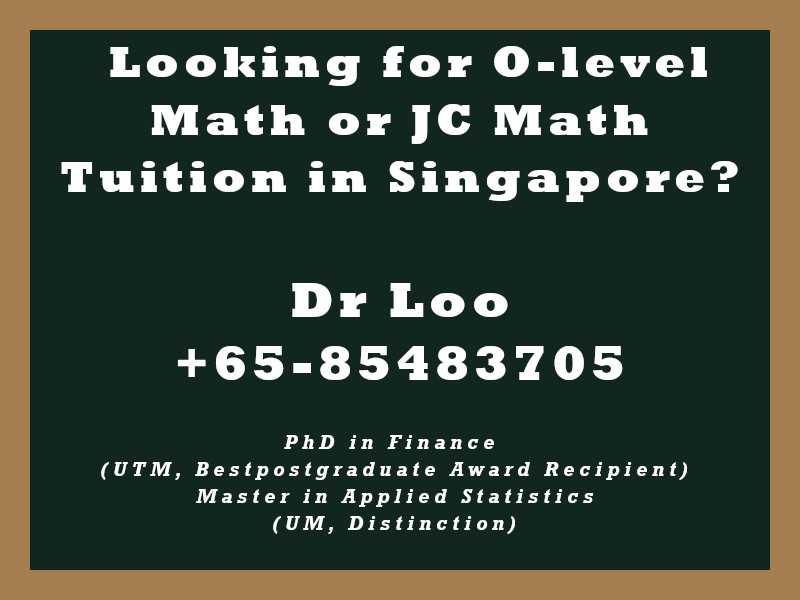+65-85483705

# Permutation & Combination

When learning the concept of permutation and combination in JC H2 Math Classes, you will come across some interesting concepts like the number of arrangement of objects in a line. In addition, you will learn how to calculate the number of arrangement of objects in circle. These concept will also take into account of cases like repetition and restriction.

## Permutation Formula

n!/(n-r)!
This formula calculates the number of permutations of n things taken r at a time

## Combination Formula

n!/[(n-r)!r!]
This formula calculates the number of combinations of n things taken r at a time

## Number of permutations of n things when there are n1, n2, …, nk repeated items.

n!/(n1!n2!…nk!)

Question

There are few letters, namely “GIIILK”.

1. How many different ways the words can be arranged in a row?
2. How many ways of arrangement that all three letters of “I” are together?

1. 20
2. 6

## About the Singapore Math Tutor - Dr Loo

I am a PhD holder with 9 years of teaching experience on teaching quantitative subjects at secondary school and university levels. My expertise are mathematics, statistics, econometrics, finance and machine learning.

Currently I do provide consultation and tuition for math tuition in Singapore. If you are looking for secondary math tutor in Singapore, JC H2 math tutor or statistics tutor in Singapore, please feel free to contact me at +65-85483705 (SMS/Whatsapp).

Among the Singapore math tuition that I provide, this includes Singapore secondary math tuition (E-math tuition & A-math tuition) and also JC Math Tuition (H1 Math Tuition & H2 Math Tuition). On the other hand, my statistics tuition in Singapore mostly focus on pre-university level until postgraduate level. For those who are looking for online tutoring service, the online O-level math tuition and online A-level math tuition are also available.## For those who are looking for math tution in Singapore

Need help with this topic? I do provide mathematics home tuition in Singapore for O-level math and also JC H2 math. In addition, online math tutoring is available as well. Feel free to contact me if you would like to know further.